Solutions to Sample First Exam        MAT 131, Fall 1998

The midterm will be held on Monday, October 19 at 8:30 pm. Be sure to bring your Stony Brook ID card and your calculator.

This sample represents the type of questions which will be on the midterm. The actual midterm will have a slightly different format, different questions, and may cover slightly different material. Just because something isn't asked here doesn't mean you aren't responsible for it. Note also that this exam may take you somewhat longer to complete than the actual exam.

1. Consider the function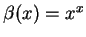. Write a limit which represents the value its derivative at x=2. Use your calculator to estimate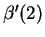. Be sure to show your work in a clear and understandable manner.

Solution:
By definition,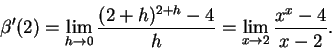So, to approximate this, we pick several points tending towards 2, and evaluate the difference quotient at each one:

 x 1.99 1.999 1.9999 2.0001 2.001 2.01 xx 3.93294 3.99323 3.99932 4.00068 4.00678 4.0684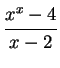6.70573 6.76586 6.77192 6.77326 6.77933 6.8404

This approximately describes a straight line -6.695224 + 6.733987 x. Plugging in at x=2, we get 6.772749 as our estimate.

(In fact, the derivative is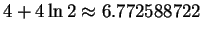, so our estimate isn't too bad).

2. In the paragraph below is a description of how the water level in a tub W(t) varied with time. Use it to draw the graph of W(t). Be sure to label any important points, and pay attention to slope, concavity, etc.

The tub held about 50 gallons of green, brackish water, with some stuff floating in it that I didn't even want to guess about. I had to get it out of there. When I opened the drain the water drained out rapidly at first, but then it went slower and slower, until it stopped completely after about 5 minutes. The tub was about 1/4-full of that nasty stuff. Would I have to stick my hand in it? Ick-- there was no way I could do that. I just stared at it for a couple of minutes, but then I got an idea. I dumped in about 10 gallons of boiling water. That did something: there was this tremendous noise like BLUUUUURP, and then the tub drained steadily, emptying completely in just a minute or so. Now all I had to do was sterilize the stupid thing before mom got home. Hmmm... should I use Lysol or gasoline?

Solution:
A graph something like the one below agrees with the description. The graph starts out at
50, then decreases slower and slower'', (which is another way of saying it is decreasing and concave up) until it finally flattens out at about 5 minutes with a value of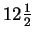. The spike'' at around 7 minutes corresponds to when the 10 gallons of boiling water were added, raising the amount to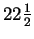, and then the level drops with constant slope, hitting the axis just about a minute later.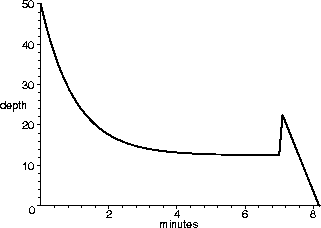3. The graph of a function f(x) is given below. Use it to draw the graphs of the functions g(x)=f(-x), h(x)=f-1(x), and k(x)=2-f(x).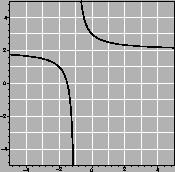Solution: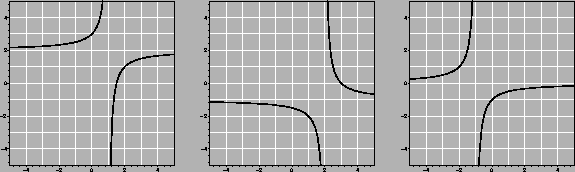4. Compute the limits: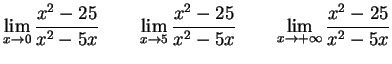Solution: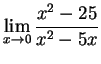does not exist. The numerator tends to 25, while the denominator tends to 0. Since the denominator is positive for x<0 and negative if x>0, the limit from below (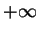) is not equal to the limit from above (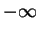), so the limit cannot be defined.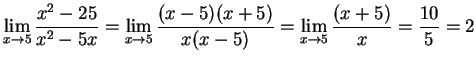.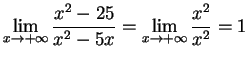.

5. Write the equation of the line tangent to the function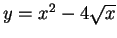at the point (4,8).

Solution:
First, we note that, so at the point (4,8), the slope is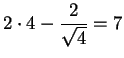. This means the line is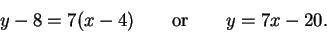6. A box without a top is to be made from 5 rectangular sheets of metal. If the bottom must be square and the box must have a volume of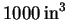, what should the dimensions be in order to minimize the amount of metal needed?

Solution:
Since we are making the box from sheets of metal, if we minimize the surface area of the box, we will minimize the amount of metal needed.

Let the base be an x by x square, and the sides be x by h. The volume of the box is h x2, so if the volume is to be 1000, we must have h = 1000/x2.

This means the surface area is given by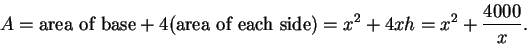We want to find the minimum of A-- to do this, we locate the stationary points of A by determining where the derivative is zero.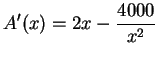, so A'(x)=0 when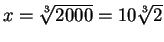. This is certainly a minimum, since it is the only positive critical point and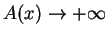as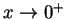and as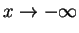.

Thus, the minimum occurs for box with dimensions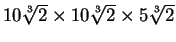, or approximately 12.6" by 12.6" by 6.3". This requires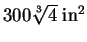(about 476.22) of sheet metal.

7. The graph of a function f(x) is given below at the left, with a function g(x) in the middle. On the right, sketch the graph of the composition f(g(x)).

Solution: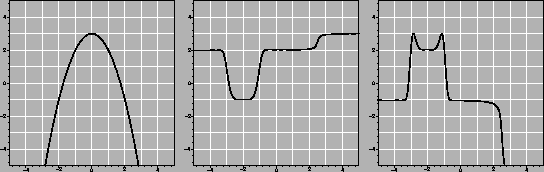In order to figure out the above, notice that on the interval [-5, -3.25], g is nearly constant at 2, and f(2)=-1. So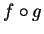is constantly -1 over this region. Similarly, f(g([-2.5,-1.5])) = f(-1) = 2 and f(g([-.75,2])) = f(2) = -1. Between these intervals, notice that g(x) covers the interval [-1, 2]. Since f(0)=3, the graph ofmust go all the way up to 3 on these sections, making Batman's ears. finally, g takes [2,3] to [2,3], which fstrecthes across [-1,-5], making Batman's shoulder. (Note that g([3,5])=3, which f sends off the graph.)

8. Sketch the graph of the derivative of the function g(x)from the previous problem.

Solution: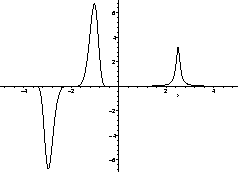9. In the table below, values for three functions are given, rounded to three decimal places. Determine whether each is of the form ax+b, ax2+b, or Cax. Then give the correct formula for f(x), g(x), and h(x).

 x 1 2 3 4 f(x) 0.21 1.725 4.25 7.785 g(x) 6.462 8.573 10.684 12.795 h(x) 2.76 3.312 3.974 4.769

Solution:
Notice that
g(2)-g(1) = g(3)-g(2) = g(4)-g(3) = 2.111, so g is the linear function, and a=2.111. So then g(1) = 2.111 +b =6.462. Consequently,

g(x) = 2.111 x + 4.351 .

Note also that h(2)/h(1) = h(3)/h(2) = h(4)/h(3) = 1.2, so h is the exponential function, with constant a = 1.2. Since h(1) = C a1 = 2.760, we must have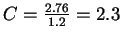. So our exponential function is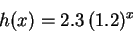Finally, we probably have f(x) = a x2 + b. If so, then f(1) = a + b = .210 and f(2) = 4a + b = 1.725. This means that f(2) - f(1) = 3a = 1.515, so a=.505, and b=-.295. This gives

f(x) = .505x2 -.295

Just to be sure, we should check another value: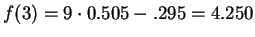. Yep.

10. Write h(x) = 3x4 + x2 + 1 as a composition of two functions f and g.

Solution:
Note that
h(x) = 3(x2)2 + x2 + 1. If we take g(x)=x2 and f(x) = 3x2 + x + 1, then f(g(x)) = h(x).

11. Use the graph of f(x) below to compute each of the following limits. If a limit does not exist, explain why.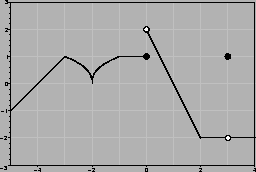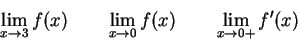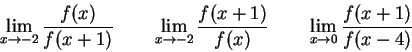Solution: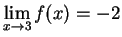.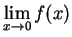does not exist, since it is 2from above and 1 from below.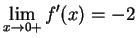, because just above 0, the graph of f is a straight line, of slope -2.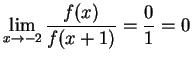.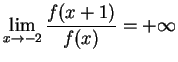, because the numerator remains near 1, while the denominator gets arbitrarily close to 0, but is always positive.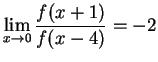. To see this, note that for x small, f(x+1) = -2x and f(x-4)=x. This means our limit becomes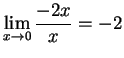.

12. Below is the graph of the derivative of a function f. The graph of f(x) is not shown. Use the graph to answer the following questions.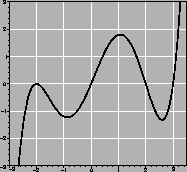a.
For what set of x-values is f decreasing?

Solution:
f will be decreasing whenever f' < 0, which happens for x<0 and 2<x<3.

b.
For what set of x-values is the graph of f concave up?

Solution:
f is concave up whenever f''>0, that is, when f' is increasing. This happens for x<-2, -1.2 < x <1.1, and 2.6 < x.

c.
Where does f have a local maximum, if any?

Solution:
f'(2)=0, and f goes from increasing to decreasing there.

d.
List all the inflection points of f, to the nearest 0.5.

Solution:
These occur at the stationary points of
f', which are, approximately, -2, -1.2, 1.1, and 2.6.

e.
If f(0)=4, which of the following is the most reasonable estimate of f(-2): 2, 4, 6, or 8?

Solution:
The best of these is
6. To see this, note that f is decreasing over this interval, with -1.2 < f'(x) < 0. If f'(x) were constantly -1.2, we would have f(-2) = 4 + 1.2(2) = 6.4, and if the slope were zero, we would have f(-2) = f(0) = 4. Since the slope is strictly between these two choices, we must have 4<f(-2)<6.4. So 6 is the best choice.

13. What is the natural domain of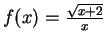?

Solution:
The largest possible domain for
f(x) isor 0 < x

Scott Sutherland
1998-10-19